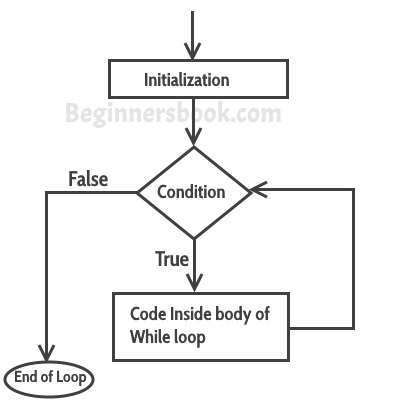World's most popular travel blog for travel bloggers.

# Loop Control Statement : While Loop

, ,

## How while Loop works?

In while loop, condition is evaluated first and if it returns true then the statements inside while loop execute. When condition returns false, the control comes out of loop and jumps to the next statement after while loop.
Note: The important point to note when using while loop is that we need to use increment or decrement statement inside while loop so that the loop variable gets changed on each iteration, and at some point condition returns false. This way we can end the execution of while loop otherwise the loop would execute indefinitely.## Simple while loop example

```class WhileLoopExample {
public static void main(String args[]){
int i=10;
while(i>1){
System.out.println(i);
i--;
}
}
}```
Output:
```10
9
8
7
6
5
4
3
2```

## Infinite while loop

```class WhileLoopExample2 {
public static void main(String args[]){
int i=10;
while(i>1)
{
System.out.println(i);
i++;
}
}
}```
This loop would never end, its an infinite while loop. This is because condition is i>1 which would always be true as we are incrementing the value of i inside while loop.
Here is another example of infinite while loop:
```while (true){
statement(s);
}```